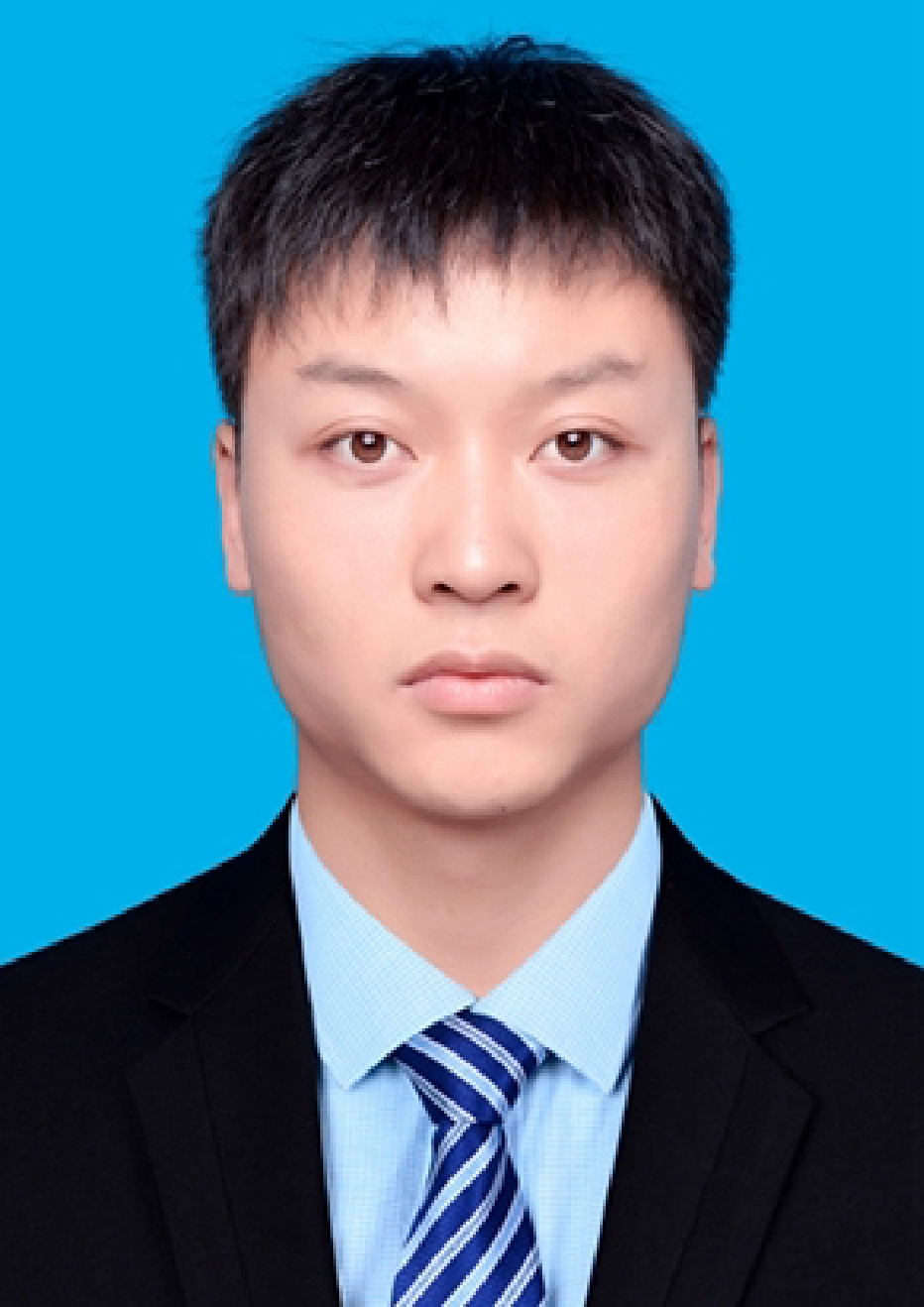# C++宏定义

+关注继续查看
C++ 宏定义将一个标识符定义为一个字符串，源程序中的该标识符均以指定的字符串来代替。预处理命令不同于一般C++语句。因此预处理命令后通常不加分号。这并不是说所有的预处理命令后都不能有分号出现。由于宏定义只是用宏名对一个字符串进行简单的替换，因此如果在宏定义命令后加了分号，将会连同分号一起进行置换。
#include <iostream>
#include <cstdlib>

using namespace std;

// 1.字符串替换
#define PI 3.1415926
// 2.宏调用函数
void f0(){cout<<0<<endl;}
#define EXP() f0
// 类似DECLARE_MESSAGE_MAP()
// 3.带参数宏
int f1(int n,int i){return n + i;}
int f2(int n,int i){return n * i;}
#define FUNC(a,b) f1(a,b) + f2(a,b)
// 类似DECLARE_DYNAMIC(CListCtrlEdit)
// 类似IMPLEMENT_DYNAMIC(CListCtrlEdit,CEdit)

void main()
{
cout<<PI<<endl;
EXP();
cout<<FUNC(1,2)<<endl;
system("pause");
}

url: http://greatverve.cnblogs.com/archive/2012/11/18/macro.html

C语言宏定义-无参数宏定义

### 9.1.1无参宏定义

#define  标识符  字符串

#define M (y*y+3*y)

【例9.1】
#define M (y*y+3*y)
main(){
int s,y;
printf("input a number:  ");
scanf("%d",&y);
s=3*M+4*M+5*M;
printf("s=%d\n",s);
}

s=3*(y*y+3*y)+4*(y*y+3*y)+5*(y*y+3*y);

#difine M y*y+3*y

s=3*y*y+3*y+4*y*y+3*y+5*y*y+3*y；

3y2+3y+4y2+3y+5y2+3y；

1. 宏定义是用宏名来表示一个字符串，在宏展开时又以该字符串取代宏名，这只是一种简单的代换，字符串中可以含任何字符，可以是常数，也可以是表达式，预处理程序对它不作任何检查。如有错误，只能在编译已被宏展开后的源程序时发现。
2. 宏定义不是说明或语句，在行末不必加分号，如加上分号则连分号也一起置换。
3. 宏定义必须写在函数之外，其作用域为宏定义命令起到源程序结束。如要终止其作用域可使用# undef命令。

#define PI 3.14159
main()
{
……
}
#undef PI

f1()
{
……
}

1. 宏名在源程序中若用引号括起来，则预处理程序不对其作宏代换。
【例9.2】
#define OK 100
main()
{
printf("OK");
printf("\n");
}

1. 宏定义允许嵌套，在宏定义的字符串中可以使用已经定义的宏名。在宏展开时由预处理程序层层代换。

#define PI 3.1415926
#define S PI*y*y          /* PI是已定义的宏名*/

printf("%f",S);

printf("%f",3.1415926*y*y);
1. 习惯上宏名用大写字母表示，以便于与变量区别。但也允许用小写字母。
2. 可用宏定义表示数据类型，使书写方便。

#define STU struct stu

STU body,*p;
#define INTEGER int

INTEGER a,b;

#define PIN1 int *
typedef (int *) PIN2;

PIN1 a,b;在宏代换后变成:
int *a,b;

PIN2 a,b;

1. 对“输出格式”作宏定义，可以减少书写麻烦。
【例9.3】中就采用了这种方法。
#define P printf
#define D "%d\n"
#define F "%f\n"
main(){
int a=5, c=8, e=11;
float b=3.8, d=9.7, f=21.08;
P(D F,a,b);
P(D F,c,d);
P(D F,e,f);
}

C语言宏定义-带参数宏定义

### 9.1.1带参宏定义

Ｃ语言允许宏带有参数。在宏定义中的参数称为形式参数，在宏调用中的参数称为实际参数。对带参数的宏，在调用中，不仅要宏展开，而且要用实参去代换形参。

#define  宏名(形参表)  字符串

宏名(实参表)；

#define M(y) y*y+3*y      /*宏定义*/
……
k=M(5);                   /*宏调用*/
……

k=5*5+3*5

【例9.4】
#define MAX(a,b) (a>b)?a:b
main(){
int x,y,max;
printf("input two numbers:    ");
scanf("%d%d",&x,&y);
max=MAX(x,y);
printf("max=%d\n",max);
}

max=(x>y)?x:y;

1. 带参宏定义中，宏名和形参表之间不能有空格出现。

#define MAX(a,b) (a>b)?a:b

#define MAX  (a,b)  (a>b)?a:b

max=MAX(x,y);

max=(a,b)(a>b)?a:b(x,y);

2. 在带参宏定义中，形式参数不分配内存单元，因此不必作类型定义。而宏调用中的实参有具体的值。要用它们去代换形参，因此必须作类型说明。这是与函数中的情况不同的。在函数中，形参和实参是两个不同的量，各有自己的作用域，调用时要把实参值赋予形参，进行“值传递”。而在带参宏中，只是符号代换，不存在值传递的问题。
3. 在宏定义中的形参是标识符，而宏调用中的实参可以是表达式。

【例9.5】
#define SQ(y) (y)*(y)
main(){
int a,sq;
printf("input a number:    ");
scanf("%d",&a);
sq=SQ(a+1);
printf("sq=%d\n",sq);
}

sq=(a+1)*(a+1);

4. 在宏定义中，字符串内的形参通常要用括号括起来以避免出错。在上例中的宏定义中(y)*(y)表达式的y都用括号括起来，因此结果是正确的。如果去掉括号，把程序改为以下形式：

【例9.6】
#define SQ(y) y*y
main(){
int a,sq;
printf("input a number:    ");
scanf("%d",&a);
sq=SQ(a+1);
printf("sq=%d\n",sq);
}

input a number:3
sq=7

sq=a+1*a+1;

【例9.7】
#define SQ(y) (y)*(y)
main(){
int a,sq;
printf("input a number:    ");
scanf("%d",&a);
sq=160/SQ(a+1);
printf("sq=%d\n",sq);
}

sq=160/SQ(a+1);

input a number:3
sq=160

sq=160/(a+1)*(a+1);
a为3时，由于“/”和“*”运算符优先级和结合性相同，则先作160/(3+1)得40，再作40*(3+1)最后得160。为了得到正确答案应在宏定义中的整个字符串外加括号，程序修改如下：

【例9.8】
#define SQ(y) ((y)*(y))
main(){
int a,sq;
printf("input a number:    ");
scanf("%d",&a);
sq=160/SQ(a+1);
printf("sq=%d\n",sq);
}

【例9.9】
main(){
int i=1;
while(i<=5)
printf("%d\n",SQ(i++));
}
SQ(int y)
{
return((y)*(y));
}

【例9.10】
#define SQ(y) ((y)*(y))
main(){
int i=1;
while(i<=5)
printf("%d\n",SQ(i++));
}

【例9.11】
#define SSSV(s1,s2,s3,v) s1=l*w;s2=l*h;s3=w*h;v=w*l*h;
main(){
int l=3,w=4,h=5,sa,sb,sc,vv;
SSSV(sa,sb,sc,vv);
printf("sa=%d\nsb=%d\nsc=%d\nvv=%d\n",sa,sb,sc,vv);
}

本文转自wenglabs博客园博客，原文链接：http://www.cnblogs.com/greatverve/archive/2012/11/18/macro.html，如需转载请自行联系原作者c++简单定义
c++简单定义
40 027 01014 0858 0733 0@自定义注解

826 0<ol start="1" class="dp-objc" style="padding:0px; border:none; list-style-position:initial; color:rgb(92,92,92); font-family:Consolas,'Courier New',Courier,mono,serif; line-height:26px; margin:0px
1054 013、宏定义中的#,##
#,## 1、在一个预处理器宏中的参数前面使用一个#,预处理器会把这个参数转换为一个字符数组。（原文：When you put a # before an argument in a preprocessor macro, the preprocessor turns that argument into a character array.
530 0
3402

0